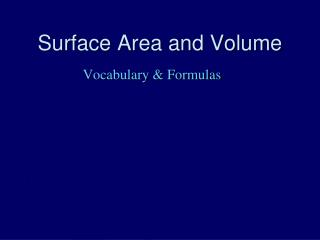DownloadDownload PresentationSurface Area and Volume

# Surface Area and Volume

Télécharger la présentation## Surface Area and Volume

- - - - - - - - - - - - - - - - - - - - - - - - - - - E N D - - - - - - - - - - - - - - - - - - - - - - - - - - -
##### Presentation Transcript

1. Surface Area and Volume Vocabulary & Formulas

2. Prism Definition: A three-dimensional solid that has two congruent and parallel faces that are polygons. The remaining faces are rectangles. Prisms are named by their faces.

3. Rectangular Prism Definition: A three-dimensional solid that has two congruent and parallel faces that are rectangles. The remaining faces are rectangles.

4. Cube Definition: A rectangular prism in which all faces are congruent squares.

5. Surface Area Definition: The sum of the areas of all of the faces of a three-dimensional figure. Ex. How much construction paper will I need to fit on the outside of the shape?

6. Volume Definition: The measure in cubic units of the interior of a solid figure; or the space enclosed by a solid figure. Ex. How much sand will it hold?

7. Surface Area of a Rectangular Prism Ex: How much construction paper would I need to fit on the outside of a particular rectangular prism? Formula: S.A. = 2LW + 2Lh + 2Wh

8. Surface Area of a Cube Ex: How much construction paper would I need to fit on the outside of a particular cube? Formula: S.A. = 6s2

9. Volume of a Rectangular Prism Ex: How much sand would I need to fill the inside of a particular rectangular prism? Formula: V = L*W*h

10. Volume of a Cube Ex: How much sand would I need to fill the inside of a particular cube? Formula: V = s3